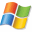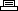Site Search

 Home: Game Cheats: PC GamesPolice Quest: SWAT 2Printer Friendly Version

```Line of sight:

Type los during game play to toggle the line of sight, allowing all
characters to be visible.

Extra money:

Sell the hand guns of the personnel that are not being used in the
mission. Since a hand gun costs \$600.00, close to \$30,000 can be
obtained for future mission or other purchases.

Mission select:

Note: This procedure involves editing a game file; create a backup copy
of the file before proceeding. Use a text editor to edit the "swat2.ini"
file in the "swat2" directory. Add the following entries under the
"[Missions Played]" header to access any mission under "Quick Play".

SM1=1
SM2=1
SM3=1
SM4=1
SM5=1
SM6=1
SM7=1
SM8=1
SM9=1
SM10=1
SM11=1
SM12=1
SM13=1
SM14=1
SM15=1
TM16=1
TM17=1
TM18=1
TM19=1
TM20=1
TM21=1
TM22=1
TM23=1
TM24=1
TM25=1
TM26=1
TM27=1
TM28=1
TM29=1
TM30=1
```